# 9 Modeling Our World Linear Modeling Copyright 2015

• Slides: 18
Download presentation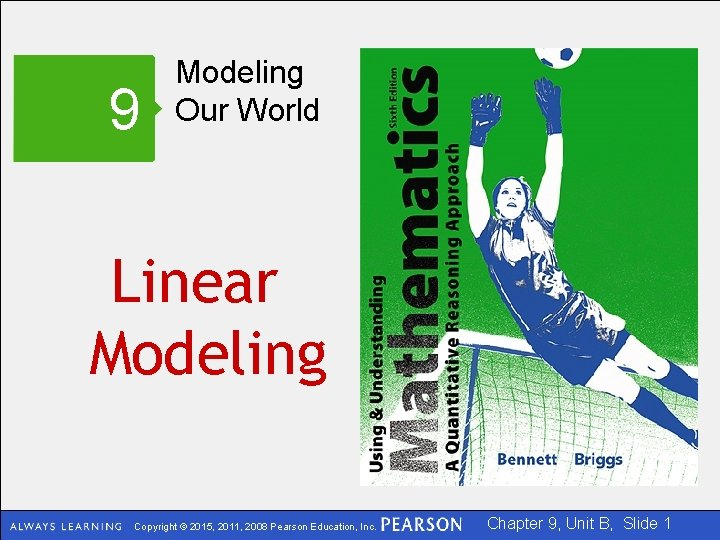9 Modeling Our World Linear Modeling Copyright © 2015, 2011, 2008 Pearson Education, Inc. Chapter 9, Unit B, Slide 1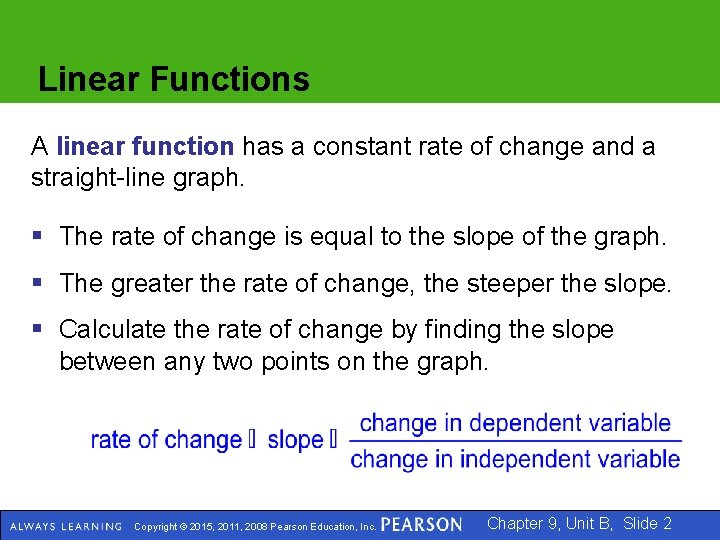Linear Functions A linear function has a constant rate of change and a straight-line graph. § The rate of change is equal to the slope of the graph. § The greater the rate of change, the steeper the slope. § Calculate the rate of change by finding the slope between any two points on the graph. Copyright © 2015, 2011, 2008 Pearson Education, Inc. Chapter 9, Unit B, Slide 2Finding the Slope of a Line To find the slope of a straight line, look at any two points and divide the change in the dependent variable by the change in the independent variable. Copyright © 2015, 2011, 2008 Pearson Education, Inc. Chapter 9, Unit B, Slide 3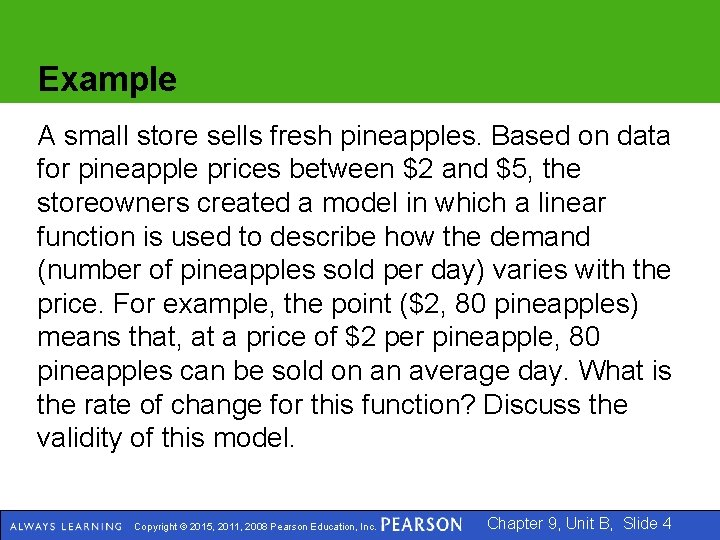Example A small store sells fresh pineapples. Based on data for pineapple prices between \$2 and \$5, the storeowners created a model in which a linear function is used to describe how the demand (number of pineapples sold per day) varies with the price. For example, the point (\$2, 80 pineapples) means that, at a price of \$2 per pineapple, 80 pineapples can be sold on an average day. What is the rate of change for this function? Discuss the validity of this model. Copyright © 2015, 2011, 2008 Pearson Education, Inc. Chapter 9, Unit B, Slide 4Example (cont) Solution Point 1 (\$2, 80) Point 2 (\$5, 50) Change in demand is 50 – 80 = – 30 The change in demand is negative because demand decreases from Point 1 to Point 2. Copyright © 2015, 2011, 2008 Pearson Education, Inc. Chapter 9, Unit B, Slide 5Example (cont) For every dollar that the price increases, the number of pineapples sold decreases by 10. Copyright © 2015, 2011, 2008 Pearson Education, Inc. Chapter 9, Unit B, Slide 6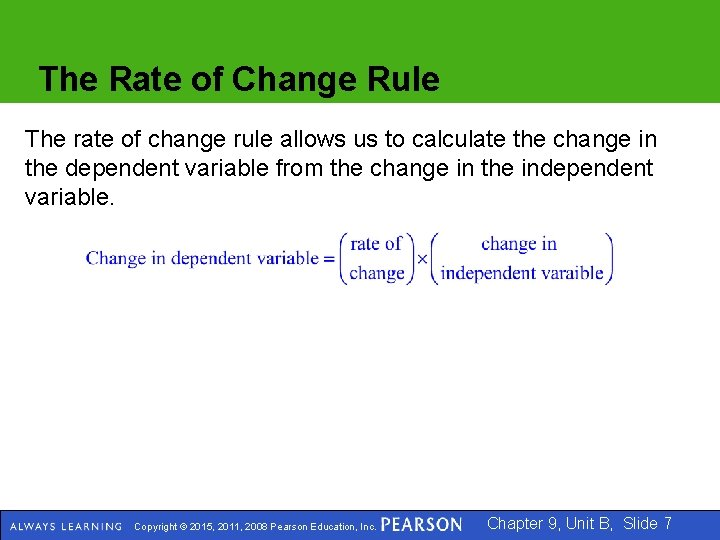The Rate of Change Rule The rate of change rule allows us to calculate the change in the dependent variable from the change in the independent variable. Copyright © 2015, 2011, 2008 Pearson Education, Inc. Chapter 9, Unit B, Slide 7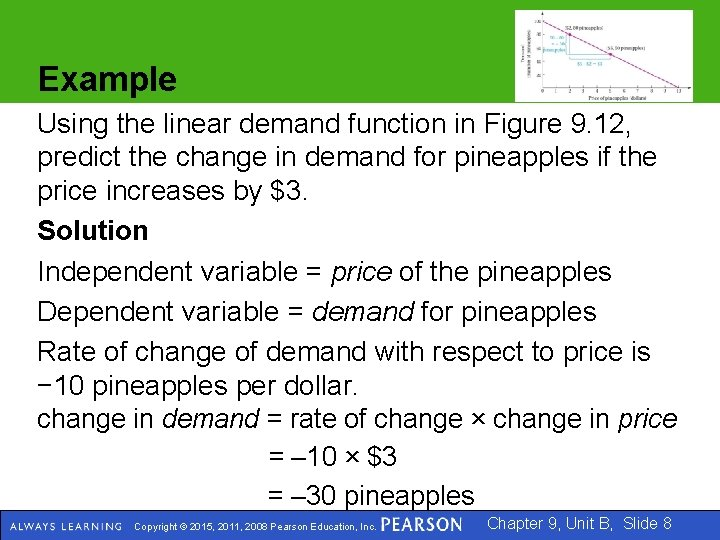Example Using the linear demand function in Figure 9. 12, predict the change in demand for pineapples if the price increases by \$3. Solution Independent variable = price of the pineapples Dependent variable = demand for pineapples Rate of change of demand with respect to price is − 10 pineapples per dollar. change in demand = rate of change × change in price = – 10 × \$3 = – 30 pineapples Copyright © 2015, 2011, 2008 Pearson Education, Inc. Chapter 9, Unit B, Slide 8Equations of Lines General Equation for a Linear Function dependent variable = initial value + (rate of change independent variable) Algebraic Equation of a Line In algebra, x is commonly used for the independent variable and y for the dependent variable. For a straight line, the slope is usually denoted by m and the initial value, or y-intercept, is denoted by b. With these symbols, the equation for a linear function becomes y = mx + b. Copyright © 2015, 2011, 2008 Pearson Education, Inc. Chapter 9, Unit B, Slide 9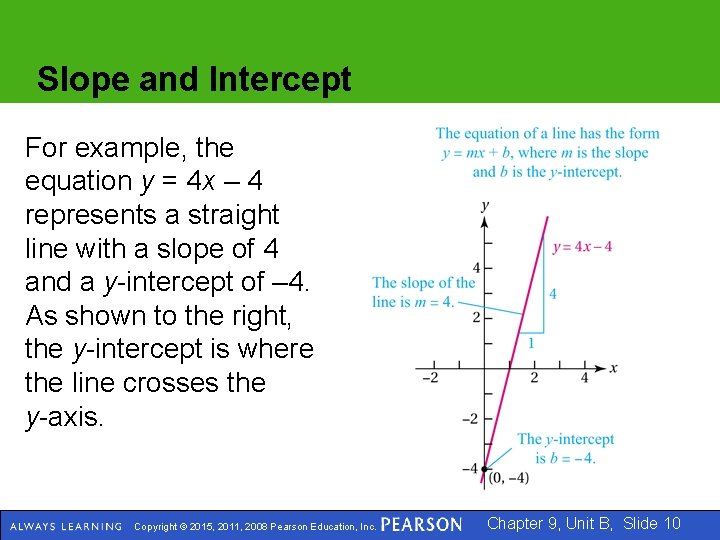Slope and Intercept For example, the equation y = 4 x – 4 represents a straight line with a slope of 4 and a y-intercept of – 4. As shown to the right, the y-intercept is where the line crosses the y-axis. Copyright © 2015, 2011, 2008 Pearson Education, Inc. Chapter 9, Unit B, Slide 10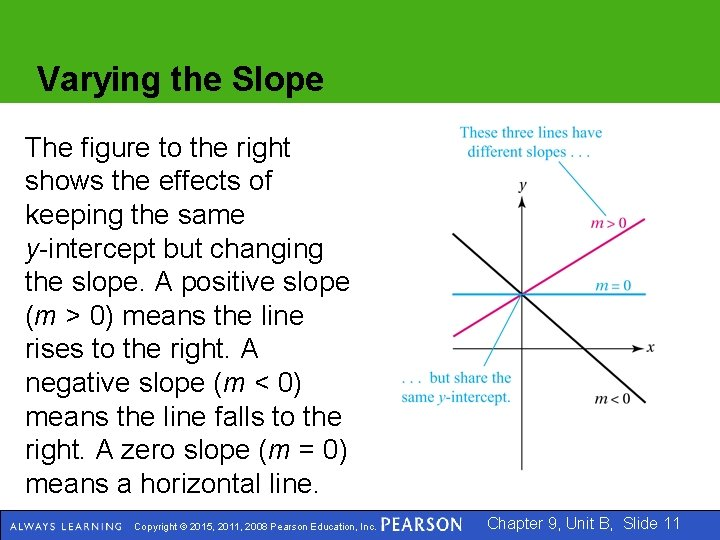Varying the Slope The figure to the right shows the effects of keeping the same y-intercept but changing the slope. A positive slope (m > 0) means the line rises to the right. A negative slope (m < 0) means the line falls to the right. A zero slope (m = 0) means a horizontal line. Copyright © 2015, 2011, 2008 Pearson Education, Inc. Chapter 9, Unit B, Slide 11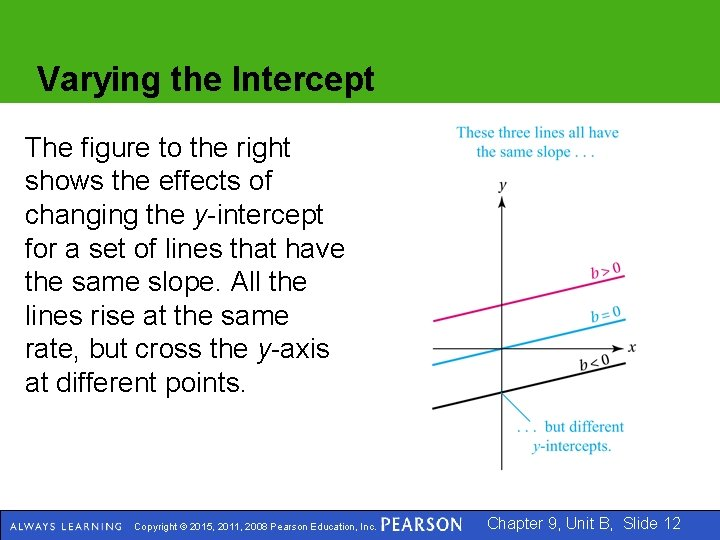Varying the Intercept The figure to the right shows the effects of changing the y-intercept for a set of lines that have the same slope. All the lines rise at the same rate, but cross the y-axis at different points. Copyright © 2015, 2011, 2008 Pearson Education, Inc. Chapter 9, Unit B, Slide 12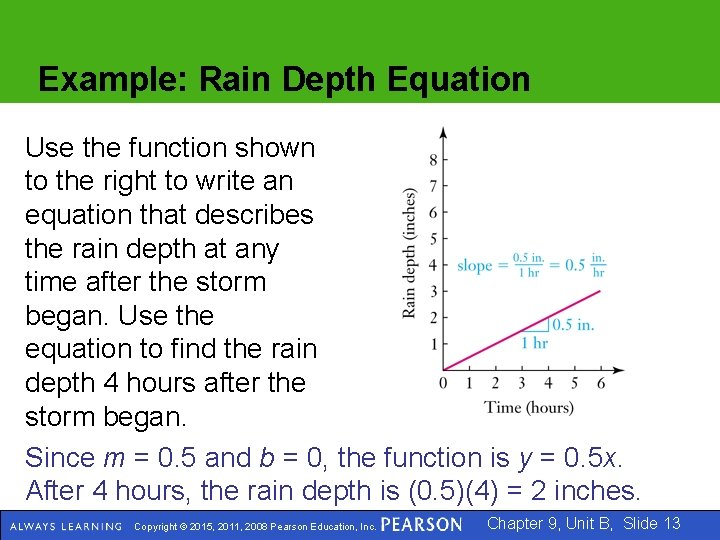Example: Rain Depth Equation Use the function shown to the right to write an equation that describes the rain depth at any time after the storm began. Use the equation to find the rain depth 4 hours after the storm began. Since m = 0. 5 and b = 0, the function is y = 0. 5 x. After 4 hours, the rain depth is (0. 5)(4) = 2 inches. Copyright © 2015, 2011, 2008 Pearson Education, Inc. Chapter 9, Unit B, Slide 13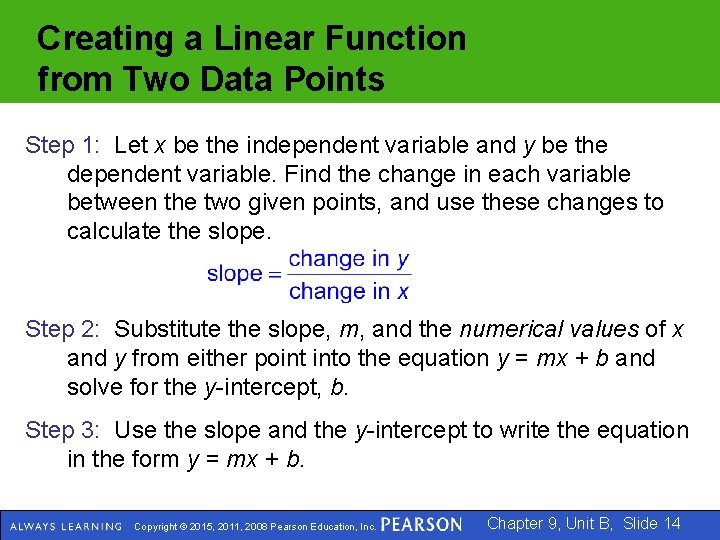Creating a Linear Function from Two Data Points Step 1: Let x be the independent variable and y be the dependent variable. Find the change in each variable between the two given points, and use these changes to calculate the slope. Step 2: Substitute the slope, m, and the numerical values of x and y from either point into the equation y = mx + b and solve for the y-intercept, b. Step 3: Use the slope and the y-intercept to write the equation in the form y = mx + b. Copyright © 2015, 2011, 2008 Pearson Education, Inc. Chapter 9, Unit B, Slide 14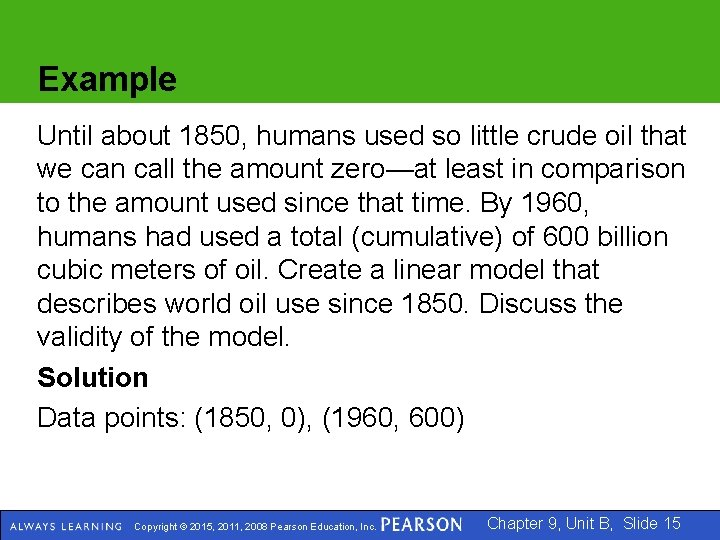Example Until about 1850, humans used so little crude oil that we can call the amount zero—at least in comparison to the amount used since that time. By 1960, humans had used a total (cumulative) of 600 billion cubic meters of oil. Create a linear model that describes world oil use since 1850. Discuss the validity of the model. Solution Data points: (1850, 0), (1960, 600) Copyright © 2015, 2011, 2008 Pearson Education, Inc. Chapter 9, Unit B, Slide 15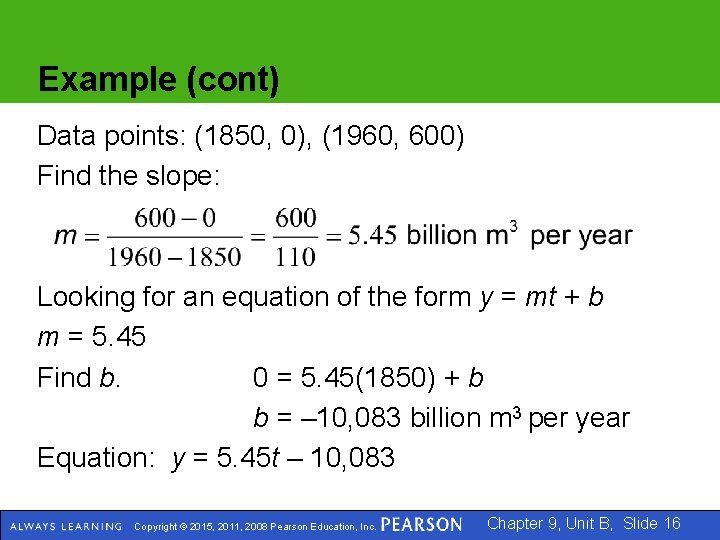Example (cont) Data points: (1850, 0), (1960, 600) Find the slope: Looking for an equation of the form y = mt + b m = 5. 45 Find b. 0 = 5. 45(1850) + b b = – 10, 083 billion m 3 per year Equation: y = 5. 45 t – 10, 083 Copyright © 2015, 2011, 2008 Pearson Education, Inc. Chapter 9, Unit B, Slide 16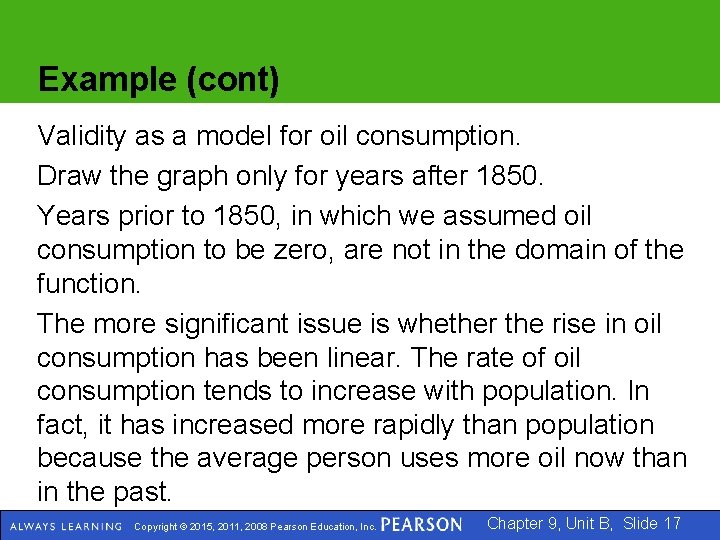Example (cont) Validity as a model for oil consumption. Draw the graph only for years after 1850. Years prior to 1850, in which we assumed oil consumption to be zero, are not in the domain of the function. The more significant issue is whether the rise in oil consumption has been linear. The rate of oil consumption tends to increase with population. In fact, it has increased more rapidly than population because the average person uses more oil now than in the past. Copyright © 2015, 2011, 2008 Pearson Education, Inc. Chapter 9, Unit B, Slide 17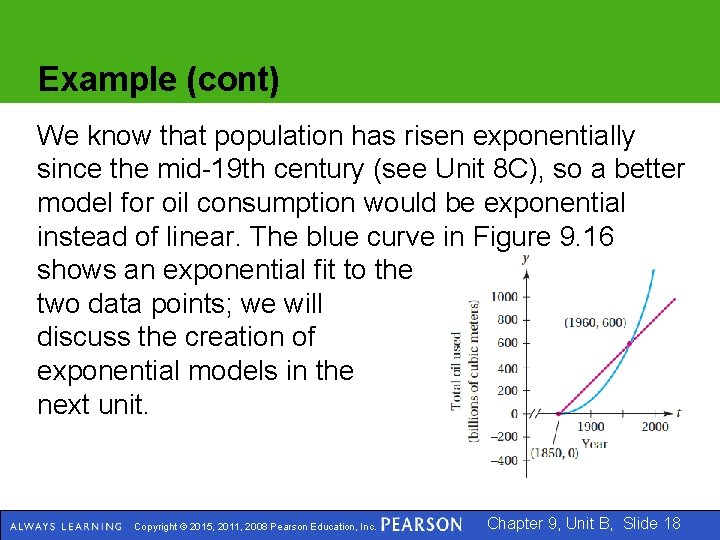Example (cont) We know that population has risen exponentially since the mid-19 th century (see Unit 8 C), so a better model for oil consumption would be exponential instead of linear. The blue curve in Figure 9. 16 shows an exponential fit to the two data points; we will discuss the creation of exponential models in the next unit. Copyright © 2015, 2011, 2008 Pearson Education, Inc. Chapter 9, Unit B, Slide 18